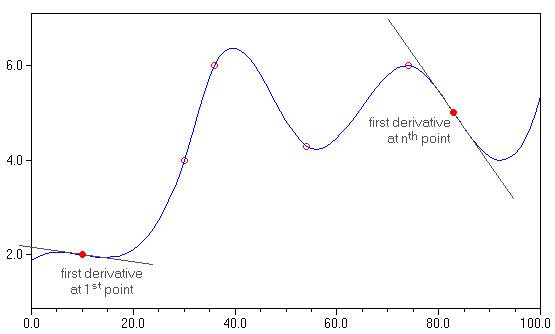The SDL Component Suite is an industry leading collection of components supporting scientific and engineering computing. Please visit the SDL Web site for more information....## CubicSplineUnit: SDL_math2 Class: Declaration: function CubicSpline (x: double): double ;

The cubic spline interpolation is a method to interpolate a function y = f(x) within a given interval. The resulting function runs through the known data points, and has a smooth first derivative and a continuous second derivative. In order to fully determine the system the following conditions have to be known:
• n data points (given by pairs of x and y coordinates), sorted by increasing x values
• the value of the first derivative at the first data point, and
• the value of the first derivative at the last data point.

As an alternative condition the second derivative at either endpoint of the interval may be required to be zero. In this case the first derivative is set by the algorithm (natural spline).The function CubicSpline calculates the value of the spline function (the blue line in the figure above) at the argument x using the data points as specified by EnterStatValue.

 Hint: Please note that the execution speed of the method CubicSpline may be much lower for the first calculation after changing any of the parameters, since the changing of a parameter triggers the solving of a set of NumData equations.

 Example: This method is used in the following example program (see http://www.lohninger.com/examples.html for downloading the code): spline

Last Update: 2018-Oct-23